# Fundamental forms of a surface

The general name for the quadratic differential forms of the surface given in coordinates on the surface and satisfying the usual transformation laws under transformations of these coordinates. The fundamental forms of a surface characterize the basic intrinsic properties of the surface and the way it is located in space in a neighbourhood of a given point; one usually singles out the so-called first, second and third fundamental forms.

The first fundamental form characterizes the interior geometry of the surface in a neighbourhood of a given point. This means that measurements on the surface can be carried out by means of it. Suppose that the surface is given by the equation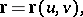whereandare coordinates on the surface; and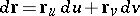is the differential of the radius vector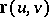along a chosen direction from a pointto an infinitesimally close point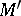(see Fig. a).Figure: f042200a

The principal linear part of growth of the arc length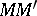is expressed by the square of: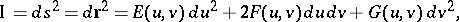where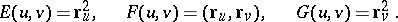The formis the first fundamental form of the surface. See also first fundamental form of a surface.

The second fundamental form characterizes the local structure of the surface in a neighbourhood of a regular point. Thus, choosea unit normal vector to the surface at, where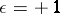if the triple of vectorshas a right-hand orientation and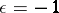in the opposite case. The doubled principal linear partof the deviation of the pointon the surface (see Fig. b) from the tangent plane at the pointis given by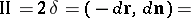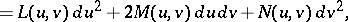where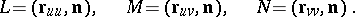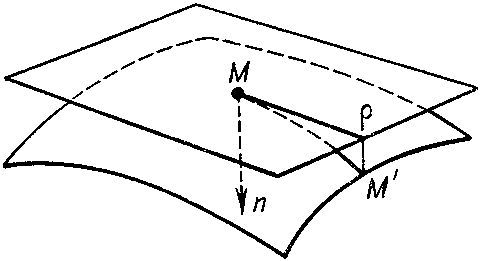Figure: f042200b

The form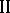is called the second fundamental form of the surface. See also Second fundamental form.

The first and second fundamental forms define two important common scalar quantities which are invariant under a transformation of the coordinates on the surface. Namely, the determinant of the ratio of the second with respect to the first one is the Gaussian curvature of the surface at the point: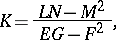while the trace of this ratio,defines the mean curvature of the surface at the point.

Specifying the first (positive definite) and second fundamental forms defines the surface up to a motion (the Bonnet theorem).

The third fundamental form of the surface is the square of the differential of the unit normal vector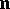to the surface at the point(see Fig. c):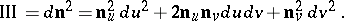Figure: f042200c

The third fundamental form of a surface is equal to the principal linear part of growth of the angle between the vectorsandunder displacement along the surface fromto; it is the first fundamental form of the spherical image of the surface (cf. Spherical map).

The three fundamental forms are related by the linear dependence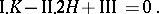In addition to the fundamental forms listed above, other fundamental forms are sometimes encountered (see, for example, ).